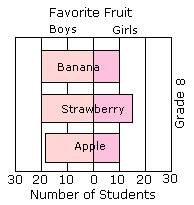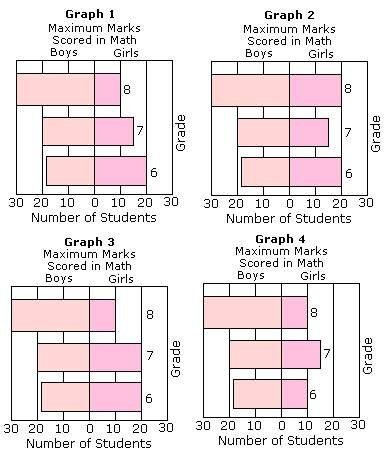## Definition Of Sliding Bar Graph

Sliding bar graphs show two categories as bars graphed in opposite directions. They can be used to compare amounts or frequencies.

### Example of Sliding Bar Graph

The sliding bar graph shown below represents the favorite fruit of grade 8 for both boys and girls.### Solved Example on Sliding Bar Graph

#### Ques: The table shows the maximum marks scored by the Grade 6, 7, and 8 students in math. Which of the following is the correct sliding bar graph for the table shown?A. Graph 1
B. Graph 2
C. Graph 3
D. Graph 4